• [TensorFlow] 交叉熵损失函数，加权交叉熵损失函数
千次阅读
2021-08-22 17:10:41

## 写在前面

在文章[TensorFlow] argmax, softmax_cross_entropy_with_logits, sparse_softmax_cross_entropy_with_logits函数详解中，提到了交叉熵损失函数的计算方式以及tensorflow中的输入和输出等。本篇文章会更细地讲一下tensorflow中交叉熵损失函数的应用，以及在优化过程中可能用到加权交叉熵损失函数的使用方式。

## 一、基础计算

当存在多个类别时，通常使用交叉熵损失函数来衡量模型的效果，也是对模型调参的重要依据，交叉熵损失函数的公式为：
L = 1 N ∑ i L i = − 1 N ∑ i ∑ C = 1 M y i c l o g P i c \begin{aligned} L = \frac{1}{N}\sum_{i}L_i = -\frac{1}{N} \sum_{i} \sum_{C=1}^My_{ic}logP_{ic} \end{aligned}
代码：

import tensorflow as tf
import numpy as np

sess=tf.Session()
#logits代表wx+b的输出，注意：logits没有进行softmax
logits = np.array([[1, 2, 3],
[4, 5, 6],
[7, 10, 3],
[8, 2, 0],
[9, 6, 3]], dtype=np.float32)
#labels是[2,2,1,0,1]的ont-hot编码形式
labels = np.array([[0, 0, 1],
[0, 0, 1],
[0, 1, 0],
[1, 0, 0],
[0, 1, 0]], dtype=np.float32)

softmax_out=tf.nn.softmax(logits)
print("softmax_out is::")
print(sess.run(softmax_out))

print("labels * tf.log(softmax_out) is::")
print(sess.run(labels * tf.log(softmax_out)))   # label和softmax_out取log后相乘

print("cross_entropy1 is::")
cross_entropy1 = -tf.reduce_sum(labels * tf.log(softmax_out), axis=1)  # 每个样本的不同label，求损失和
print(sess.run(cross_entropy1))


结果：

softmax_out is::
[[9.0030573e-02 2.4472848e-01 6.6524094e-01]
[9.0030573e-02 2.4472848e-01 6.6524094e-01]
[4.7384717e-02 9.5174748e-01 8.6788135e-04]
[9.9719369e-01 2.4717962e-03 3.3452120e-04]
[9.5033026e-01 4.7314156e-02 2.3556333e-03]]
labels * tf.log(softmax_out) is::
[[-0.0000000e+00 -0.0000000e+00 -4.0760601e-01]
[-0.0000000e+00 -0.0000000e+00 -4.0760601e-01]
[-0.0000000e+00 -4.9455535e-02 -0.0000000e+00]
[-2.8102510e-03 -0.0000000e+00 -0.0000000e+00]
[-0.0000000e+00 -3.0509458e+00 -0.0000000e+00]]
cross_entropy1 is::
[4.0760601e-01 4.0760601e-01 4.9455535e-02 2.8102510e-03 3.0509458e+00]


## 二、tf.nn.softmax_cross_entropy_with_logits和tf.nn.sparse_softmax_cross_entropy_with_logits

1.两个函数的输出结果相同，区别在于输入的labels不同。
2.对于sparse_softmax_cross_entropy_with_logits， labels的size是[batch_size]，每个label的取值范围是[0, num_classes-1]，即每个样本的label就是0、1、2.
3.对于softmax_cross_entropy_with_logits, labels的size是[batch_size, num_classes]，即sparse_softmax_cross_entropy_with_logits中labels的one-hot值。

代码：

import tensorflow as tf
import numpy as np

sess = tf.Session()
# logits代表wx+b的输出，注意：logits没有进行softmax
logits = np.array([[1, 2, 3],
[4, 5, 6],
[7, 10, 3],
[8, 2, 0],
[9, 6, 3]], dtype=np.float32)

print("cross_entropy2 is::")
cross_entropy2 = tf.nn.softmax_cross_entropy_with_logits(logits=logits, labels=labels)
print(sess.run(cross_entropy2))

# labels是[2,2,1,0,1]的ont-hot编码形式
labels = np.array([[0, 0, 1],
[0, 0, 1],
[0, 1, 0],
[1, 0, 0],
[0, 1, 0]], dtype=np.float32)

print("cross_entropy3 is::")
classes = tf.argmax(labels, axis=1)  # array([2,2,1,0,1])
cross_entropy3 = tf.nn.sparse_softmax_cross_entropy_with_logits(logits=logits, labels=classes)
print(sess.run(cross_entropy3))


结果：

cross_entropy2 is::
[4.0760595e-01 4.0760595e-01 4.9455538e-02 2.8102214e-03 3.0509458e+00]
cross_entropy3 is::
[4.0760595e-01 4.0760595e-01 4.9455538e-02 2.8102214e-03 3.0509458e+00]


## 三、tf.losses.softmax_cross_entropy 和 tf.losses.sparse_softmax_cross_entropy

1.主要用于进行不同样本的loss计算，但可通过权重来控制loss损失值
2.默认weights=1，等价于tf.reduce_mean(tf.nn.softmax_cross_entropy_with_logits)
4.weights为向量时，算出的每个loss需要乘以对应样本权重，再求均值
5.tf.losses.sparse_softmax_cross_entropy 同理等等价于tf.reduce_mean(tf.nn.sparse_softmax_cross_entropy_with_logits),只不过输入labels是非one-hot编码格式

代码：

import tensorflow as tf
import numpy as np
sess = tf.Session()
#logits代表wx+b的输出，注意：logits没有进行softmax
logits = np.array([[1, 2, 3],
[4, 5, 6],
[7, 10, 3],
[8, 2, 0],
[9, 6, 3]], dtype=np.float32)
#labels是[2,2,1,0,1]的ont-hot编码形式
labels = np.array([[0, 0, 1],
[0, 0, 1],
[0, 1, 0],
[1, 0, 0],
[0, 1, 0]], dtype=np.float32)

cross1 = tf.nn.softmax_cross_entropy_with_logits(labels=labels,logits=logits)
cross2 = tf.losses.softmax_cross_entropy(onehot_labels=labels, logits=logits)
cross3 = tf.losses.softmax_cross_entropy(onehot_labels=labels, logits=logits, weights=0.3)

print("cross1 is::")
print (sess.run(cross1))
print("cross2 is::")
print (sess.run(cross2))
print("tf.reduce_mean(cross1) is::")
print (sess.run(tf.reduce_mean(cross1)))

print("cross3 is::")
print (sess.run(cross3))
print("0.3*tf.reduce_mean(cross1) is::")
print (sess.run(0.3*tf.reduce_mean(cross1)))


结果：

cross1 is::
[4.0760595e-01 4.0760595e-01 4.9455538e-02 2.8102214e-03 3.0509458e+00]
cross2 is::
0.7836847
tf.reduce_mean(cross1) is::
0.7836847


## 四、加权交叉熵损失 – 样本加权

tf.losses.softmax_cross_entropy可以对损失进行加权，当每个样本的权重不同时，可以按照样本的权重加权。

import tensorflow as tf
import numpy as np
sess = tf.Session()
#logits代表wx+b的输出，注意：logits没有进行softmax
logits = np.array([[1, 2, 3],
[4, 5, 6],
[7, 10, 3],
[8, 2, 0],
[9, 6, 3]], dtype=np.float32)
#labels是[2,2,1,0,1]的ont-hot编码形式
labels = np.array([[0, 0, 1],
[0, 0, 1],
[0, 1, 0],
[1, 0, 0],
[0, 1, 0]], dtype=np.float32)

cross2 = tf.losses.softmax_cross_entropy(onehot_labels=labels, logits=logits)
cross3 = tf.losses.softmax_cross_entropy(onehot_labels=labels, logits=logits, weights=0.3)    # 每个样本都权重0.3

print("cross2 is::")
print (sess.run(cross2))
print("cross3 is::")
print (sess.run(cross3))
print("0.3*tf.reduce_mean(cross1) is::")
print (sess.run(0.3*tf.reduce_mean(cross1)))

cross4 = tf.losses.softmax_cross_entropy(onehot_labels=labels, logits=logits,weights=[1, 2, 3, 4, 5])  # 用于控制每个样本的权重
print("cross4 is::", cross4)
print (sess.run(cross4))
print("sum", 4.0760595e-01 * 1 + 4.0760595e-01 * 2 + 4.9455538e-02 * 3 + 2.8102214e-03 * 4 + 3.0509458e+00 * 5)
print("average", (4.0760595e-01 * 1 + 4.0760595e-01 * 2 + 4.9455538e-02 * 3 + 2.8102214e-03 * 4 + 3.0509458e+00 * 5)/5.)


结果：

cross2 is::
0.7836847
cross3 is::
0.23510543
0.3*tf.reduce_mean(cross1) is::
0.23510541
cross4 is::
3.3274307
sum 16.6371543496
average 3.3274308699199997


## 五、加权交叉熵损失 – 类别加权

实际可能会遇到这样的情况，对于每个样本是没有权重的，但是针对不同的类别，有相应的权重，比如，类别3更不希望错误，所以类别3的损失权重可能会设置的比其他大。

对于我们需要重视的类别，可以给其较高的权重，（权重越高，损失越大，模型越会学好这个类别），如：
某个类别较少，则可以给出较高的权重，使其训练的更好
某个类别不允许错误，则需要尽量训练好这个数据，可以将其权重调高

#logits代表wx+b的输出，注意：logits没有进行softmax
logits = np.array([[1, 2, 3],
[4, 5, 6],
[7, 10, 3],
[8, 2, 0],
[9, 6, 3]], dtype=np.float32)
#labels是[2,2,1,0,1]的ont-hot编码形式
labels = np.array([[0, 0, 1],
[0, 0, 1],
[0, 1, 0],
[1, 0, 0],
[0, 1, 0]], dtype=np.float32)

class_weights = tf.constant([[1.0, 1.5, 4.0]])  # 3个类别的权重，用于控制每个类别的权重
weights = tf.reduce_sum(class_weights * labels, axis=1)  # 向量化的权重
print("weights is::", sess.run(weights))

cross1 = tf.nn.softmax_cross_entropy_with_logits(labels=labels,logits=logits)
print("cross1 is::")
print(sess.run(cross1))
weighted_losses = cross1 * weights
print("weighted_losses is::")
print(sess.run(weighted_losses))
print("tf.reduce_mean(weighted_losses) is::")
print(sess.run(tf.reduce_mean(weighted_losses)))

softmax_out=tf.nn.softmax(logits)
print("softmax_out is::")
print(sess.run(softmax_out))
print("labels * tf.log(softmax_out) is::")
print(sess.run(labels * tf.log(softmax_out)))   # label和softmax_out取log后相乘

sum = 4.0760601e-01 * 4 + 4.0760601e-01 * 4 + 4.9455535e-02 * 1.5 + 2.8102510e-03 * 1 + 3.0509458e+00 * 1.5   # 通过普通的计算方法看下是否与结果一致
print("sum/5 is::", sum/5)


结果：

weights is:: [4.  4.  1.5 1.  1.5]
cross1 is::
[4.0760595e-01 4.0760595e-01 4.9455538e-02 2.8102214e-03 3.0509458e+00]
weighted_losses is::
[1.6304238e+00 1.6304238e+00 7.4183308e-02 2.8102214e-03 4.5764189e+00]
tf.reduce_mean(weighted_losses) is::
1.582852
softmax_out is::
[[9.0030573e-02 2.4472848e-01 6.6524094e-01]
[9.0030573e-02 2.4472848e-01 6.6524094e-01]
[4.7384717e-02 9.5174748e-01 8.6788135e-04]
[9.9719369e-01 2.4717962e-03 3.3452120e-04]
[9.5033026e-01 4.7314156e-02 2.3556333e-03]]
labels * tf.log(softmax_out) is::
[[-0.0000000e+00 -0.0000000e+00 -4.0760601e-01]
[-0.0000000e+00 -0.0000000e+00 -4.0760601e-01]
[-0.0000000e+00 -4.9455535e-02 -0.0000000e+00]
[-2.8102510e-03 -0.0000000e+00 -0.0000000e+00]
[-0.0000000e+00 -3.0509458e+00 -0.0000000e+00]]
sum/5 is:: 1.5828520667


## 六、加权交叉熵损失 – 类别转移矩阵加权

上面是针对不同的类别，权重不同，但是若不是针对固定的类别有权重，而是比如：将类别1预测为类别2，这种错误代价较高，反而将类别1预测为类别3代价较低，这种方式怎么来进行损失加权呢？如预测值和真实值预测权重矩阵为：

真实类别1真实类别2真实类别3
预测类别1142
预测类别2511
预测类别3431

其中 w 01 = 4 w_{01}=4 表示预测类别为1实际类别为2的权重， w 02 = 2 w_{02}=2 表示预测类别为1实际类别为3的权重。

代码：

#logits代表wx+b的输出，注意：logits没有进行softmax
logits = np.array([[1, 2, 3],
[4, 5, 6],
[7, 10, 3],
[2, 8, 0],
[9, 6, 3]], dtype=np.float32)
#labels是[2,2,1,0,1]的ont-hot编码形式
labels = np.array([[0, 1, 0],
[0, 0, 1],
[0, 1, 0],
[1, 0, 0],
[0, 1, 0]], dtype=np.float32)
transfer_weights = tf.constant([[1.0, 4.0, 2.0],
[5.0, 1.0, 1.0],
[4.0, 3.0, 1.0]])

weighted_logits = tf.matmul(logits, transfer_weights)
print("weighted_logits is::")
print(sess.run(weighted_logits))  # 得到预测值为logits情况下，实际label=1、label=2、label=3下的加权logits

softmax_out=tf.nn.softmax(weighted_logits)
print("softmax_out is::")
print(sess.run(softmax_out))
print("labels * tf.log(softmax_out) is::")
print(sess.run(labels * tf.log(softmax_out)))   # label和softmax_out取log后相乘

cross1 = tf.nn.softmax_cross_entropy_with_logits(labels=labels,logits=weighted_logits)
cross2 = tf.losses.softmax_cross_entropy(onehot_labels=labels, logits=weighted_logits)
print ("cross1 is::", sess.run(cross1))
print ("cross2 is::", sess.run(cross2))

sum = 8.000336 + 34. + 22. + 0. + 0.6931472  # 常规算法，与参数结果比较是否一致
print("average is::", sum/5.)


结果：

weighted_logits is::
[[23. 15.  7.]
[53. 39. 19.]
[69. 47. 27.]
[42. 16. 12.]
[51. 51. 27.]]
softmax_out is::
[[9.99664545e-01 3.35350080e-04 1.12497425e-07]
[9.99999166e-01 8.31528041e-07 1.71390690e-15]
[1.00000000e+00 2.78946810e-10 5.74952202e-19]
[1.00000000e+00 5.10908893e-12 9.35762291e-14]
[5.00000000e-01 5.00000000e-01 1.88756719e-11]]
labels * tf.log(softmax_out) is::
[[ -0.         -8.000336   -0.       ]
[ -0.         -0.        -34.       ]
[  0.        -22.         -0.       ]
[  0.         -0.         -0.       ]
[ -0.         -0.6931472  -0.       ]]
cross1 is:: [ 8.000336  34.        22.         0.         0.6931472]
cross2 is:: 12.938696
average is:: 12.93869664


参考文章：
Tensorflow交叉熵的代码实现tensorflow 深度学习
更多相关内容
• 交叉熵损失函数原理详解 之前在代码中经常看见交叉熵损失函数(CrossEntropy Loss)，只知道它是分类问题中经常使用的一种损失函数，对于其内部的原理总是模模糊糊，而且一般使用交叉熵作为损失函数时，在模型的输出层...
• 本文将对以下几种tensorflow中常用的交叉熵损失函数进行比较： tf.losses.sigmoid_cross_entropy tf.nn.sigmoid_cross_entropy_with_logits tf.losses.softmax_cross_entropy tf.nn.softmax_cross_entropy_with_...
• 我们会发现，在机器学习实战中，做分类问题的时候经常会使用一种损失函数（Loss Function）——交叉熵损失函数（CrossEntropy Loss）。但是，为什么在做分类问题时要用交叉熵损失函数而不用我们经常使用的平方损失...
• 论文中"Holistically-Nested Edge Detection"使用的加权损失函数，具体用法见博客http://blog.csdn.net/majinlei121/article/details/78884531
• 交叉熵代价函数(Cross-entropy cost function)是用来衡量人工神经网络(ANN)的预测值与实际值的一种方式。与二次代价函数相比，它能更有效地促进ANN的训练。在介绍交叉熵代价函数之前，本文先简要介绍二次代价函数，...

交叉熵代价函数(Cross-entropy cost function)是用来衡量人工神经网络(ANN)的预测值与实际值的一种方式。与二次代价函数相比，它能更有效地促进ANN的训练。在介绍交叉熵代价函数之前，本文先简要介绍二次代价函数，以及其存在的不足。

二次代价函数的不足

ANN的设计目的之一是为了使机器可以像人一样学习知识。人在学习分析新事物时，当发现自己犯的错误越大时，改正的力度就越大。比如投篮：当运动员发现自己的投篮方向离正确方向越远，那么他调整的投篮角度就应该越大，篮球就更容易投进篮筐。同理，我们希望：ANN在训练时，如果预测值与实际值的误差越大，那么在反向传播训练的过程中，各种参数调整的幅度就要更大，从而使训练更快收敛。然而，如果使用二次代价函数训练ANN，看到的实际效果是，如果误差越大，参数调整的幅度可能更小，训练更缓慢。

以一个神经元的二类分类训练为例，进行两次实验(ANN常用的激活函数为sigmoid函数，该实验也采用该函数)：输入一个相同的样本数据x=1.0(该样本对应的实际分类y=0)；两次实验各自随机初始化参数，从而在各自的第一次前向传播后得到不同的输出值，形成不同的代价(误差)：图片.png

实验1：第一次输出值为0.82image

实验2：第一次输出值为0.98

在实验1中，随机初始化参数，使得第一次输出值为0.82(该样本对应的实际值为0)；经过300次迭代训练后，输出值由0.82降到0.09，逼近实际值。而在实验2中，第一次输出值为0.98，同样经过300迭代训练，输出值只降到了0.20。

从两次实验的代价曲线中可以看出：实验1的代价随着训练次数增加而快速降低，但实验2的代价在一开始下降得非常缓慢；直观上看，初始的误差越大，收敛得越缓慢。

其实，误差大导致训练缓慢的原因在于使用了二次代价函数。二次代价函数的公式如下：图片.png图片.png图片.png

如图所示，实验2的初始输出值(0.98)对应的梯度明显小于实验1的输出值(0.82)，因此实验2的参数梯度下降得比实验1慢。这就是初始的代价(误差)越大，导致训练越慢的原因。与我们的期望不符，即：不能像人一样，错误越大，改正的幅度越大，从而学习得越快。图片.png图片.png

说起交叉熵损失函数「Cross Entropy Loss」，脑海中立马浮现出它的公式：image

我们已经对这个交叉熵函数非常熟悉，大多数情况下都是直接拿来使用就好。但是它是怎么来的？为什么它能表征真实样本标签和预测概率之间的差值？上面的交叉熵函数是否有其它变种？也许很多朋友还不是很清楚！没关系，接下来我将尽可能以最通俗的语言回答上面这几个问题。

1. 交叉熵损失函数的数学原理

我们知道，在二分类问题模型：例如逻辑回归「Logistic Regression」、神经网络「Neural Network」等，真实样本的标签为 [0，1]，分别表示负类和正类。模型的最后通常会经过一个 Sigmoid 函数，输出一个概率值，这个概率值反映了预测为正类的可能性：概率越大，可能性越大。

Sigmoid 函数的表达式和图形如下所示：imageimage

其中 s 是模型上一层的输出，Sigmoid 函数有这样的特点：s = 0 时，g(s) = 0.5；s >> 0 时， g ≈ 1，s << 0 时，g ≈ 0。显然，g(s) 将前一级的线性输出映射到 [0，1] 之间的数值概率上。这里的 g(s) 就是交叉熵公式中的模型预测输出 。

我们说了，预测输出即 Sigmoid 函数的输出表征了当前样本标签为 1 的概率：image

很明显，当前样本标签为 0 的概率就可以表达成：image

重点来了，如果我们从极大似然性的角度出发，把上面两种情况整合到一起：image

也即，当真实样本标签 y = 0 时，上面式子第一项就为 1，概率等式转化为：image

当真实样本标签 y = 1 时，上面式子第二项就为 1，概率等式转化为：image

两种情况下概率表达式跟之前的完全一致，只不过我们把两种情况整合在一起了。重点看一下整合之后的概率表达式，我们希望的是概率 P(y|x) 越大越好。首先，我们对 P(y|x) 引入 log 函数，因为 log 运算并不会影响函数本身的单调性。则有：image

我们希望 log P(y|x) 越大越好，反过来，只要 log P(y|x) 的负值 -log P(y|x) 越小就行了。那我们就可以引入损失函数，且令 Loss = -log P(y|x)即可。则得到损失函数为：image

非常简单，我们已经推导出了单个样本的损失函数，是如果是计算 N 个样本的总的损失函数，只要将 N 个 Loss 叠加起来就可以了：image

这样，我们已经完整地实现了交叉熵损失函数的推导过程。

2. 交叉熵损失函数的直观理解

可能会有读者说，我已经知道了交叉熵损失函数的推导过程。但是能不能从更直观的角度去理解这个表达式呢？而不是仅仅记住这个公式。好问题！接下来，我们从图形的角度，分析交叉熵函数，加深大家的理解。

首先，还是写出单个样本的交叉熵损失函数：image

我们知道，当 y = 1 时：image

这时候，L 与预测输出的关系如下图所示：image

看了 L 的图形，简单明了！横坐标是预测输出，纵坐标是交叉熵损失函数 L。显然，预测输出越接近真实样本标签 1，损失函数 L 越小；预测输出越接近 0，L 越大。因此，函数的变化趋势完全符合实际需要的情况。当 y = 0 时：image

这时候，L 与预测输出的关系如下图所示：image

同样，预测输出越接近真实样本标签 0，损失函数 L 越小；预测函数越接近 1，L 越大。函数的变化趋势也完全符合实际需要的情况。

从上面两种图，可以帮助我们对交叉熵损失函数有更直观的理解。无论真实样本标签 y 是 0 还是 1，L 都表征了预测输出与 y 的差距。

另外，重点提一点的是，从图形中我们可以发现：预测输出与 y 差得越多，L 的值越大，也就是说对当前模型的 “ 惩罚 ” 越大，而且是非线性增大，是一种类似指数增长的级别。这是由 log 函数本身的特性所决定的。这样的好处是模型会倾向于让预测输出更接近真实样本标签 y。

3. 交叉熵损失函数的其它形式

什么？交叉熵损失函数还有其它形式？没错！我刚才介绍的是一个典型的形式。接下来我将从另一个角度推导新的交叉熵损失函数。

这种形式下假设真实样本的标签为 +1 和 -1，分别表示正类和负类。有个已知的知识点是Sigmoid 函数具有如下性质：image

这个性质我们先放在这，待会有用。

好了，我们之前说了 y = +1 时，下列等式成立：image

如果 y = -1 时，并引入 Sigmoid 函数的性质，下列等式成立：image

重点来了，因为 y 取值为 +1 或 -1，可以把 y 值带入，将上面两个式子整合到一起：image

接下来，同样引入 log 函数，得到：image

要让概率最大，反过来，只要其负数最小即可。那么就可以定义相应的损失函数为：image

还记得 Sigmoid 函数的表达式吧？将 g(ys) 带入：image

好咯，L 就是我要推导的交叉熵损失函数。如果是 N 个样本，其交叉熵损失函数为：image

接下来，我们从图形化直观角度来看。当 y = +1 时：image

这时候，L 与上一层得分函数 s 的关系如下图所示：image

横坐标是 s，纵坐标是 L。显然，s 越接近真实样本标签 1，损失函数 L 越小；s 越接近 -1，L 越大。另一方面，当 y = -1 时：image

这时候，L 与上一层得分函数 s 的关系如下图所示：image

同样，s 越接近真实样本标签 -1，损失函数 L 越小；s 越接近 +1，L 越大。

4. 总结

本文主要介绍了交叉熵损失函数的数学原理和推导过程，也从不同角度介绍了交叉熵损失函数的两种形式。第一种形式在实际应用中更加常见，例如神经网络等复杂模型；第二种多用于简单的逻辑回归模型。

展开全文• 目录0. 前言1.损失函数（Loss Function）1.1 损失项1.2 正则化项2....今天在学习cs231n的时候看到了关于交叉熵损失函数的讲解，发现之前虽然经常用到这个损失函数，但却对里面的细节很模糊，学完之后更清晰了一

# 0. 前言

有段时间没写博客了，前段时间主要是在精读一些计算机视觉的论文（比如yolov1），以及学cs231n这门AI和计算机视觉领域的经典课程。我发现很多事情不能着急，质变需要量变的积累，违背事物发展的客观规律，往往适得其反。

今天在学习cs231n的时候看到了关于交叉熵损失函数的讲解，发现之前虽然经常用到这个损失函数，但却对里面的细节很模糊，学完之后更清晰了一些，所以做个总结笔记，方便以后快速回顾。学习就是不断重复的过程。

# 1.损失函数（Loss Function）

损失函数作用是：衡量真实值和模型预测值之间的差异

为什么要有损失函数呢？

以分类任务为例，在神经网络中，模型前向传播求出每一类别的得分（score），然后将score带入损失函数中，求出Loss。再使用梯度下降法，反向传播，通过降低损失值（Loss）对模型的参数进行优化。

损失函数通常由损失项正则化项组成。

## 1.1 损失项

损失项的目的就是之前说的，为了衡量真实值和模型预测值之间的差异

## 1.2 正则化项

正则化项的目的是对高次项的特征进行惩罚，减轻过拟合的程度，进而可以提高模型的泛化能力。

常用的正则化有L1正则化和L2正则化。假如现在要训练一个线性模型，y = Wx + b，其中W就是我们要训练的参数（由w1,w2,…wn n个参数组成），b是偏置项。

则L1正则化就是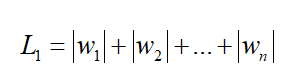L2正则化为：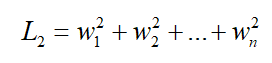# 2. 交叉熵损失函数

交叉熵损失函数是多分类问题中很常用的损失函数，而交叉熵损失函数又离不开softmax函数。因为在多分类问题中，神经网络最后一层全连接层的原始输出只是每一类的得分（score）向量。比如以MINIST手写数字识别为例，我们构建的神经网络的最后一层肯定只有10个神经元（每个神经元输出0-9数字的得分）。

注意，这里10个神经元输出的只是得分，而非该图片属于该数字的概率。那要输出概率该怎么办呢？这就要用到sofrmax处理了。

## 2.1 softmax

softmax函数定义为：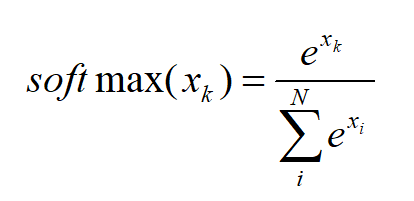比如对于一个猫cat，狗dog，马horse的三分类问题，我们的神经网络最后一层给出每类得分为[10,9, 8]，那softmax应该这么求：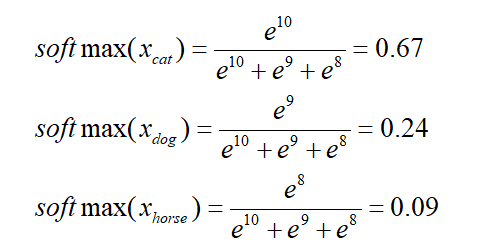表示的含义是：模型推测该图片是猫的概率为67%，狗的概率为24%，马的概率为9%

用ex求出的值恒为正，可以表示概率。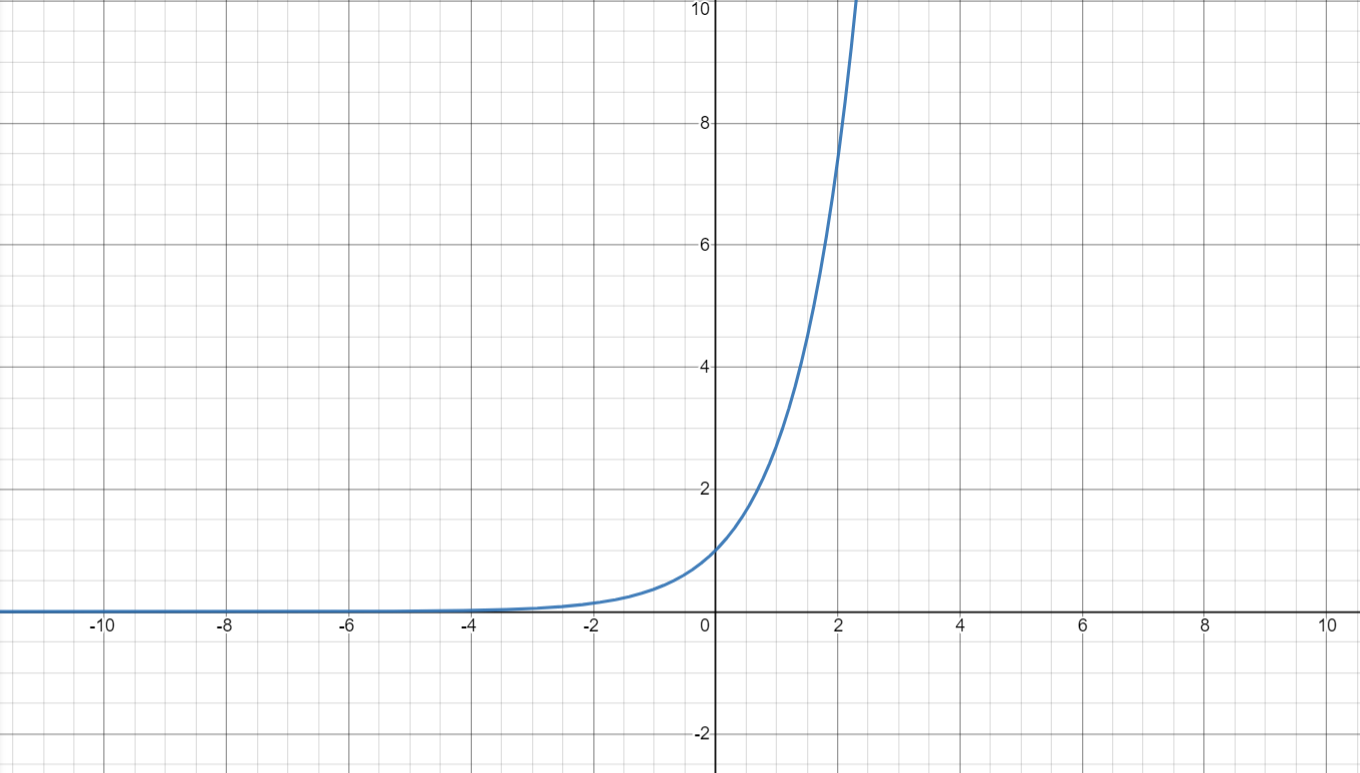另外，从上面的例子也可以看出softmax可以把得分差距拉的更大：最后的全链接层得分输出为：[10,9,8]，相差其实不大。而经过softmax之后输出为：[0.67,0.24,0.09]，相对而言差距更大了。

## 2.2 交叉熵

终于说到交叉熵了，前面说了，交叉熵损失函数离不开softmax，应该先经过softmax求出每类的概率，再求交叉熵，可是为什么要这样呢？

这得从信息论中交叉熵的定义说起。交叉熵H(p,q)的定义为：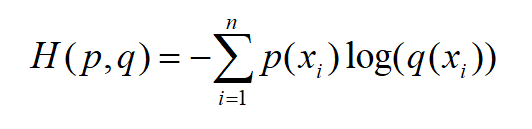注意，这里的log是以e为底的！

其中p为真实概率分布，而q为预测的概率分布。既然是概率，那肯定得满足：每个值在0-1之间，且所有值的和为1。

说到这里，我们再拐回去看softmax函数的定义：可以经过softmax层后的输出的每个值在0-1之间，且所有值的和为1。

这就是为什么在交叉熵损失函数中需要先进行softmax处理了，因为这是交叉熵本身的定义决定的（输入必须为概率）。

再来看交叉熵损失函数：在机器学习中，真实概率分布p(xi）就是训练集的标签，预测的概率分布q(xi)就是我们模型预测的结果。再以刚刚的猫、狗、马三分类为例，我们提供一张猫的照片，则真实概率分布p(xi)的向量为[1, 0, 0]，而我们模型预测的概率分布q(xi)的向量为：[0.67, 0.24, 0.09]。

则对于该预测结果的交叉熵损失函数为：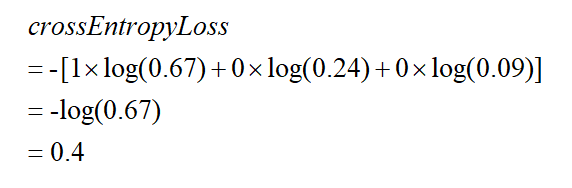-log(x)函数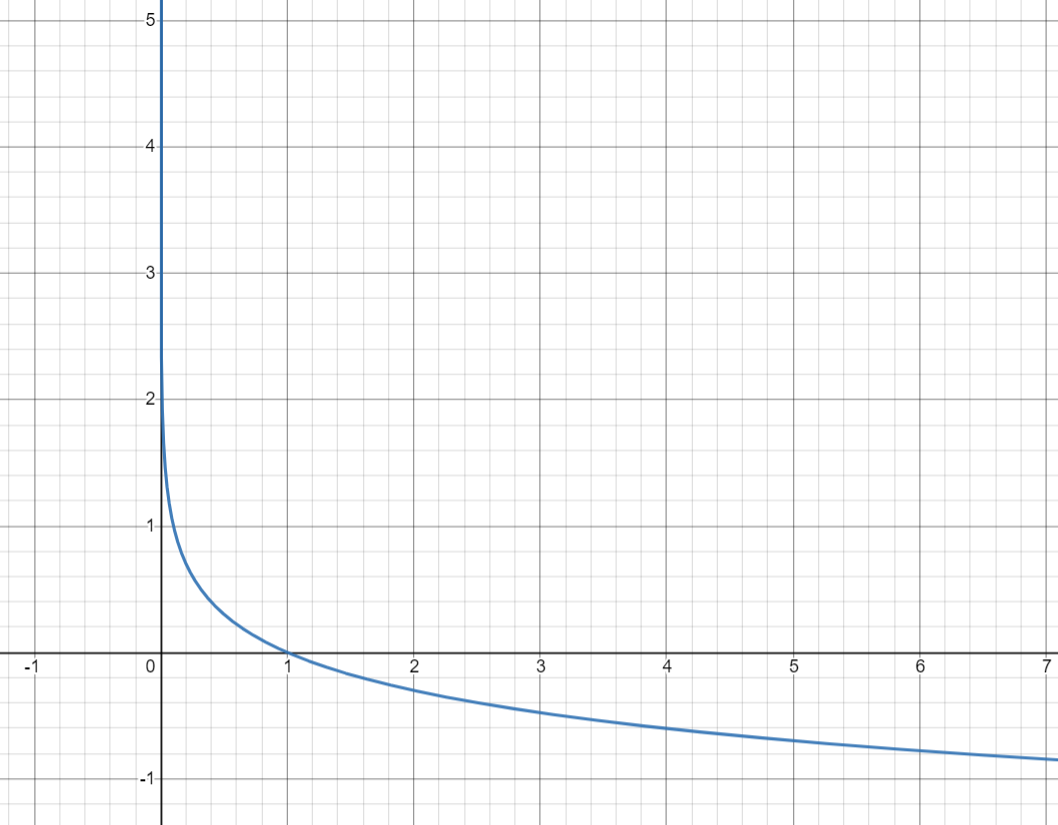因为是-log(q(x))，而q(x)是概率，在0-1之间，所以我们只看0-1之间的函数样子。

不难看出：

（1）当预测结果正确时，该正确类别的概率越大（q(x)越接近1），交叉熵损失函数越小。

（2）当预测结果正确，但正确类别的概率不够大时（q(x)较小），交叉熵损失函数较大。

（3）当预测结果错误时，交叉熵损失函数也很大。

END：）

参考

交叉熵损失函数原理详解

展开全文神经网络 机器学习 深度学习 正则化
• 在之前的课程中，我们已经完成了从0建立深层神经...接下来，我们将从梯度下降法向外拓展，介绍神经网络的损失函数、常用优化算法等信息，实现神经网络的学习和迭代。本节主要讲解神经网络常用的损失函数，并在PyTorch

## 一、机器学习中的优化思想

在之前的学习中，我们建立神经网络时总是先设定好与的值（或者由我们调用的PyTorch类帮助我们随机生成权重向量），接着通过加和求出 z z ，再在 z z 上嵌套sigmoid或者softmax函数，最终获得神经网络的输出。我们的代码及计算流程，总是从神经网络的左侧向右侧计算的。之前我们提到过，这是神经网络的正向传播过程。但很明显，这并不是神经网络算法的全流程，这个流程虽然可以输出预测结果，但却无法保证神经网络的输出结果与真实值接近。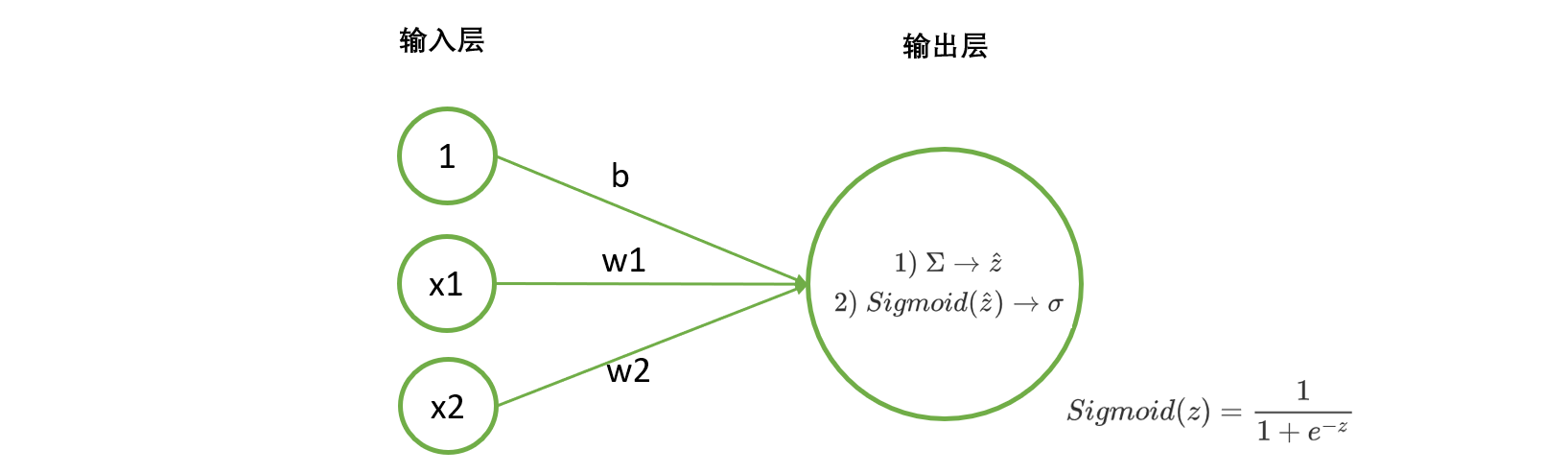在讲解线性回归时，我们提起过，线性回归的任务就是构造一个预测函数来映射输入的特征矩阵 X X 和标签值 y y 的线性关系。构造预测函数核心就是找出模型的权重向量 w w ，并令线性回归的输出结果与真实值相近，也就是求解线性方程组中的 w w b b 。对神经网络而言也是如此，我们的核心任务是求解一组最适合的 w w b b ，令神经网络的输出结果与真实值接近。找寻这个 w w b b 的过程就是“学习”，也叫做“训练”或者“建模”。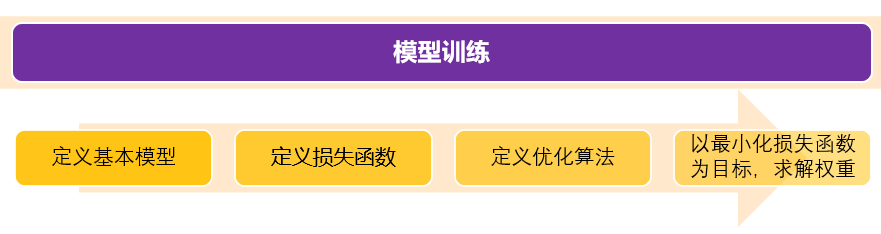1）提出基本模型，明确目标
我们的基本模型就是我们自建的神经网络架构，我们需要求解的就是神经网络架构中的权重向量 w w
2）确定损失函数/目标函数
我们需要定义某个评估指标，用以衡量模型权重为 w w 的情况下，预测结果与真实结果的差异。当真实值与预测值差异越大时，我们就认为神经网络学习过程中丢失了许多信息，丢失的这部分被形象地称为”损失“，因此评估真实值与预测值差异的函数被我们称为“损失函数”。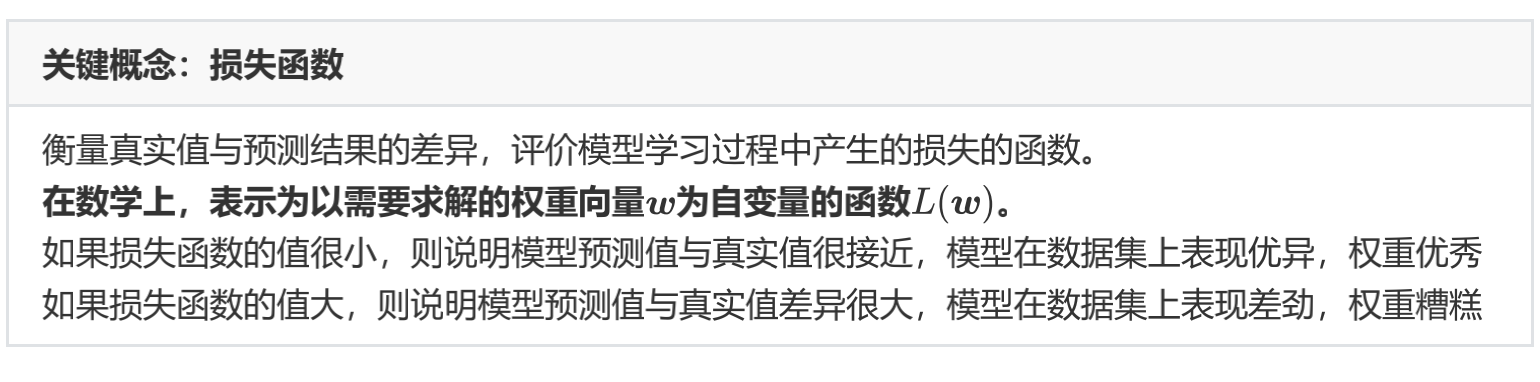我们希望损失函数越小越好，以此，我们将问题转变为求解函数 L ( w ) L(w) 的最小值所对应的自变量 w w 。但是，损失函数往往不是一个简单的函数，求解复杂函数就需要复杂的数学工具。在这里，我们使用的数学工具可能有两部分：

• 将损失函数 L ( w ) L(w) 转变成凸函数的数学方法，常见的有拉格朗日变换等
• 在凸函数上求解 L ( w ) L(w) 的最小值对应的 w w 的方法，也就是以梯度下降为代表的优化算法

3）确定适合的优化算法
4）利用优化算法，最小化损失函数，求解最佳权重（训练）
之前我们在线性回归上走过这个全流程。对线性回归，我们的损失函数是SSE，优化算法是最小二乘法和梯度下降法，两者都是对机器学习来说非常重要的优化算法。但遗憾的是，最小二乘法作为入门级优化算法，有较多的假设和先决条件，不足以应对神经网络需要被应用的各种复杂环境。梯度下降法应用广泛，不过也有很多问题需要改进。接下来，我将主要以分类深层神经网络为例来介绍神经网络中所使用的入门级损失函数及优化算法。

## 二、回归：误差平方和SSE

对于回归类神经网络而言，最常见的损失函数是SSE（Sum of the Squared Errors），现在已经是我们第三次见到SSE的公式了：
S S E = ∑ i = 1 m ( z i − z ^ i ) 2 S S E=\sum_{i=1}^{m}\left(z_{i}-\hat{z}_{i}\right)^{2}
其中 z i z_{i} 是样本 i i 的真实值，而 z ^ i \hat{z}_{i} 是样本 i i 的预测值。对于全部样本的平均损失，则可以写作：
M S E = 1 m ∑ i = 1 m ( z i − z ^ i ) 2 M S E=\frac{1}{m} \sum_{i=1}^{m}\left(z_{i}-\hat{z}_{i}\right)^{2}
在PyTorch中，我们可以简单通过以下代码调用MSE：

import torch
from torch.nn import MSELoss #类
yhat = torch.randn(size=(50,),dtype=torch.float32)
y = torch.randn(size=(50,),dtype=torch.float32)
criterion = MSELoss() #实例化
loss = criterion(yhat,y)
loss #没有设置随机数种子，所以每次运行的数字都会不一致
#tensor(1.5714)

#在MSELoss中有重要的参数，reduction
#当reduction = "mean" (默认也是mean)，则输出MSE
#当reduction = "sum"，则输出SSE
criterion = MSELoss(reduction = "mean") #实例化
criterion(yhat,y)
#tensor(1.5714)
criterion = MSELoss(reduction = "sum")
criterion(yhat,y)
#tensor(78.5707)


## 三、二分类交叉熵损失函数

在这一节中，我们将介绍二分类神经网络的损失函数：二分类交叉熵损失函数（Binary Cross Entropy Loss），也叫做对数损失（log loss）。这个损失函数被广泛地使用在任何输出结果是二分类的神经网络中，即不止限于单层神经网络，还可被拓展到多分类中，因此理解二分类交叉熵损失是非常重要的一环。大多数时候，除非特殊声明为二分类，否则提到交叉熵损失，我们会默认算法的分类目标是多分类。

二分类交叉熵损失函数是由极大似然估计推导出来的，对于有m个样本的数据集而言，在全部样本上的平均损失写作：
L ( w ) = − ∑ i = 1 m ( y i ∗ ln ⁡ ( σ i ) + ( 1 − y i ) ∗ ln ⁡ ( 1 − σ i ) ) L(w)=-\sum_{i=1}^{m}\left(y_{i} * \ln \left(\sigma_{i}\right)+\left(1-y_{i}\right) * \ln \left(1-\sigma_{i}\right)\right)
在单个样本的损失写作：
L ( w ) i = − ( y i ∗ ln ⁡ ( σ i ) + ( 1 − y i ) ∗ ln ⁡ ( 1 − σ i ) ) L(w)_{i}=-\left(y_{i} * \ln \left(\sigma_{i}\right)+\left(1-y_{i}\right) * \ln \left(1-\sigma_{i}\right)\right)
其中，ln是以自然底数 e e 为底的对数函数， w w 表示求解出来的一组权重（在等号的右侧， w w σ \sigma 里），m是样本的个数， y i y_{i} 是样本i上真实的标签， σ i \sigma_{i} 是样本i上，基于参数 w w 计算出来的sigmoid函数的返回值， x i x_{i} 是样本i各个特征的取值。我们的目标，就是求解出使 L ( w ) L(w) 最小的 w w 取值。注意，在神经网络中，特征张量 X X 是自变量，权重是 w w 。但在损失函数中，权重 w w 是损失函数的自变量，特征x和真实标签y都是已知的数据，相当于是常数。不同的函数中，自变量和参数各有不同，因此大家需要在数学计算中，尤其是求导的时候避免混淆。

### 1 极大似然估计求解二分类交叉熵损失

二分类交叉熵损失函数是怎么来的呢？为什么这个函数就能够代表二分类的时候，真实值与预测值的差异呢？

在这里，我们基于极大似然法来推导交叉熵损失，这个推导过程能够帮助我们充分了解交叉熵损失的含义，以及为什么的最小化能够实现模型在数据集上的拟合最好。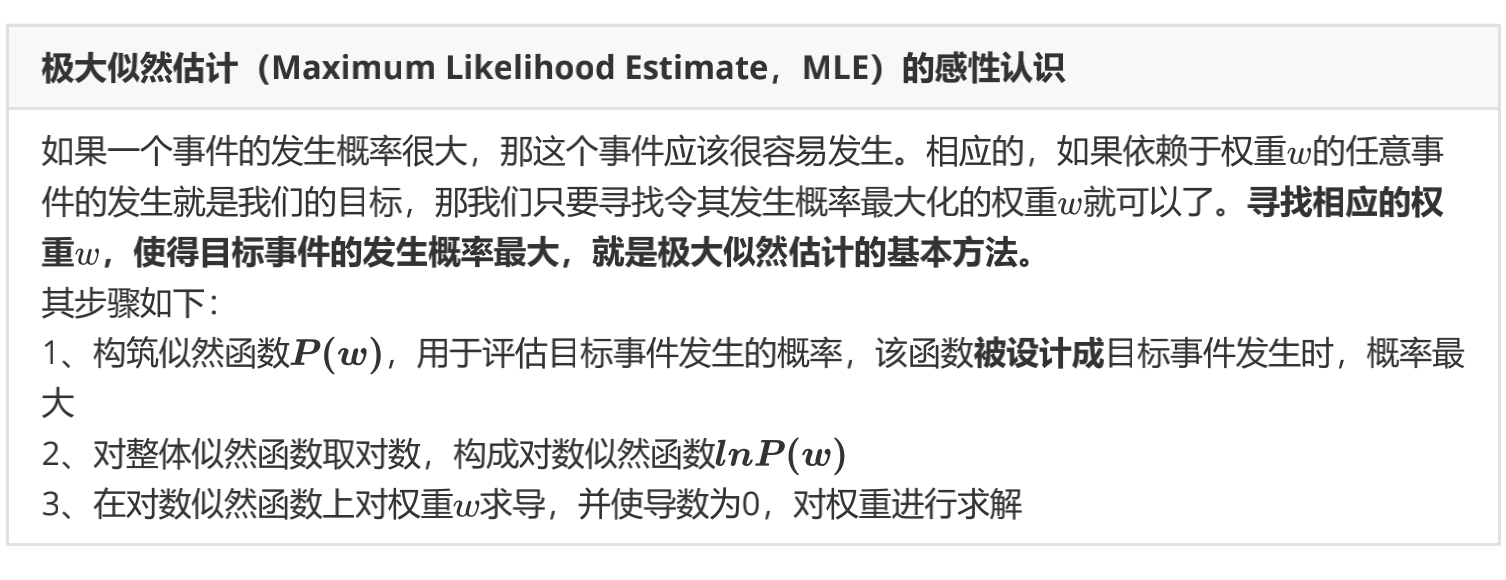在二分类的例子中，我们的“任意事件”就是每个样本的分类都正确，对数似然函数的负数就是我们的损失函数。接下来，我们来看看逻辑回归的对数似然函数是怎样构筑的。

• 构筑对数似然函数

二分类神经网络的标签是[0,1]，此标签服从伯努利分布(即0-1分布)，因此可得：

样本i在由特征向量 x i x_{i} 和权重向量 w w 组成的预测函数中，样本标签被预测为1的概率为：对二分类而言， σ \sigma 就是sigmoid函数的结果。
样本i在由特征向量 x i x_{i} 和权重向量 w w 组成的预测函数中，样本标签被预测为0的概率为：P 1 P_{1} 值为1的时候，代表样本i的标签被预测为1，当 P 0 P_{0} 的值为1的时候，代表样本i的标签被预测为0。 P 0 P_{0} P 1 P_{1} 相加是一定等于1的。

假设样本i的真实标签 y i y_{i} 为1，并且 P 1 P_{1} 也为1的话，那就说明我们将 i i 的标签预测为1的概率很大，与真实值一致，那模型的预测就是准确的，拟合程度很高，信息损失很少。相反，如果真实标签为1，我们的 P 1 P_{1} 却很接近0，这就说明我们将 i i 的标签预测为1的概率很小，即与真实值一致的概率很小，那模型的预测就是失败的，拟合程度很低，信息损失很多。当 y i y_{i} 为0时，也是同样的道理。所以，当 y i y_{i} 为1的时候，我们希望 P 1 P_{1} 非常接近1，当 y i y_{i} 为0的时候，我们希望 P 0 P_{0} 非常接近1，这样，模型的效果就很好，信息损失就很少。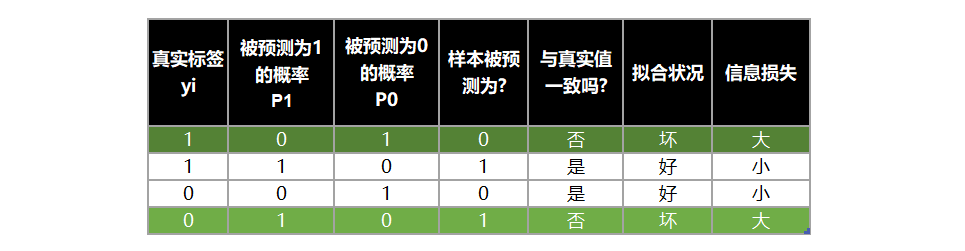将两种取值的概率整合，我们可以定义如下等式：
P ( y ^ i ∣ x i , w ) = P 1 y i ∗ P 0 1 − y i P\left(\hat{y}_{i} \mid \boldsymbol{x}_{i}, \boldsymbol{w}\right)=P_{1}^{y_{i}} * P_{0}^{1-y_{i}}
这个等式代表同时代表了 P 1 P_{1} P 0 P_{0} ，在数学上，它被叫做逻辑回归的假设函数。

当样本i的真实标签 y i y_{i} 为1的时候，1 - y i y_{i} 就等于0， P 0 P_{0} 的0次方就是1，所以 P ( y ^ i ∣ x i , w ) P\left(\hat{y}_{i} \mid \boldsymbol{x}_{i}, \boldsymbol{w}\right) 就等于 P 1 P_{1} ，这个时候，如果 P 1 P_{1} 为1，模型的效果就很好，损失就很小。

同理，当 y i y_{i} 为0的时候， P ( y ^ i ∣ x i , w ) P\left(\hat{y}_{i} \mid \boldsymbol{x}_{i}, \boldsymbol{w}\right) 就等于 P 0 P_{0} ，此时如果 P 0 P_{0} 非常接近1，模型的效果就很好，损失就很小。

所以，为了达成让模型拟合好，损失小的目的，我们每时每刻都希望 P ( y ^ i ∣ x i , w ) P\left(\hat{y}_{i} \mid \boldsymbol{x}_{i}, \boldsymbol{w}\right) 的值等于1。而 P ( y ^ i ∣ x i , w ) P\left(\hat{y}_{i} \mid \boldsymbol{x}_{i}, \boldsymbol{w}\right) 的本质是样本i由特征向量 x i x_{i} 和权重 w w 组成的预测函数中，预测出所有可能的 y ^ i \hat{y}_{i} 的概率，因此1是它的最大值。也就是说，每时每刻，我们都在追求 P ( y ^ i ∣ x i , w ) P\left(\hat{y}_{i} \mid \boldsymbol{x}_{i}, \boldsymbol{w}\right) 的最大值。而寻找相应的参数 w w ，使得每次得到的预测概率最大，正是极大似然估计的基本方法，不过 P ( y ^ i ∣ x i , w ) P\left(\hat{y}_{i} \mid \boldsymbol{x}_{i}, \boldsymbol{w}\right) 是对单个样本而言的，因此我们还需要将其拓展到多个样本上。

P ( y ^ i ∣ x i , w ) P\left(\hat{y}_{i} \mid \boldsymbol{x}_{i}, \boldsymbol{w}\right) 是对单个样本i而言的函数，对一个训练集的m个样本来说，我们可以定义如下等式来表达所有样本在特征张量 X X 和权重向量 w w 组成的预测函数中，预测出所有可能的 y ^ i \hat{y}_{i} 的概率P为：
P = ∏ i = 1 m P ( y ^ i ∣ x i , w ) = ∏ i = 1 m ( P 1 y i ∗ P 0 1 − y i ) = ∏ i = 1 m ( σ i y i ∗ ( 1 − σ i ) 1 − y i ) \begin{aligned} \boldsymbol{P} &=\prod_{i=1}^{m} P\left(\hat{y}_{i} \mid x_{i}, w\right) \\ &=\prod_{i=1}^{m}\left(P_{1}^{y_{i}} * P_{0}^{1-y_{i}}\right) \\ &=\prod_{i=1}^{m}\left(\sigma_{i}^{y_{i}} *\left(1-\sigma_{i}\right)^{1-y_{i}}\right) \end{aligned}
这个函数就是逻辑回归的似然函数。对该概率P取以e为底的对数，再由 log ⁡ ( A ∗ B ) = log ⁡ A + log ⁡ B \log (A * B)=\log A+\log B log ⁡ A B = B log ⁡ A \log A^{B}=B \log A 可得到逻辑回归的对数似然函数：
ln ⁡ P = ln ⁡ ∏ i = 1 m ( σ i y i ∗ ( 1 − σ i ) 1 − y i ) = ∑ i = 1 m ln ⁡ ( σ i y i ∗ ( 1 − σ i ) 1 − y i ) = ∑ i = 1 m ( ln ⁡ σ i y i + ln ⁡ ( 1 − σ i ) 1 − y i ) = ∑ i = 1 m ( y i ∗ ln ⁡ ( σ i ) + ( 1 − y i ) ∗ ln ⁡ ( 1 − σ i ) ) \begin{aligned} \ln \boldsymbol{P} &=\ln \prod_{i=1}^{m}\left(\sigma_{i}^{y_{i}} *\left(1-\sigma_{i}\right)^{1-y_{i}}\right) \\ &=\sum_{i=1}^{m} \ln \left(\sigma_{i}^{y_{i}} *\left(1-\sigma_{i}\right)^{1-y_{i}}\right) \\ &=\sum_{i=1}^{m}\left(\ln \sigma_{i}^{y_{i}}+\ln \left(1-\sigma_{i}\right)^{1-y_{i}}\right) \\ &=\sum_{i=1}^{m}\left(y_{i} * \ln \left(\sigma_{i}\right)+\left(1-y_{i}\right) * \ln \left(1-\sigma_{i}\right)\right) \end{aligned}
这就是我们的二分类交叉熵函数。为了数学上的便利以及更好地定义”损失”的含义，我们希望将极大值问题转换为极小值问题，因此我们对 l n P lnP 取负，并且让权重 w w 作为函数的自变量，就得到了我们的损失函数 ：
L ( w ) = − ∑ i = 1 m ( y i ∗ ln ⁡ ( σ i ) + ( 1 − y i ) ∗ ln ⁡ ( 1 − σ i ) ) L(w)=-\sum_{i=1}^{m}\left(y_{i} * \ln \left(\sigma_{i}\right)+\left(1-y_{i}\right) * \ln \left(1-\sigma_{i}\right)\right)
现在，我们已经将模型拟合中的“最小化损失”问题，转换成了对函数求解极值的问题。这就是一个，基于逻辑回归的返回值 σ i \sigma_{i} 的概率性质以及极大似然估计得出的损失函数。在这个函数上，我们只要追求最小值，就能让模型在训练数据上的拟合效果最好，损失最低

在极大似然估计中，我们只要在对数似然函数上对权重 w w 求导，再令导数为0，就可以求解出最合适的 w w ，但是对于像交叉熵这样复杂的损失函数，加上神经网络中复杂的权重组合，令所有权重的导数为0并一个个求解方程的难度很大。因此我们要使用优化算法，这部分我们下一章展开来聊。

### 2 用tensor实现二分类交叉熵损失

现在，让我们在PyTorch中来实现二分类交叉熵损失函数。首先使用基本的tensor方法来试试看，以加深我们对二分类交叉熵损失的印象：

import torch
import time
N = 3*pow(10,3)
torch.random.manual_seed(420)
X = torch.rand((N,4),dtype=torch.float32)
y = torch.randint(low=0,high=2,size=(N,1),dtype=torch.float32) #high取不到
zhat = torch.mm(X,w)
sigma = torch.sigmoid(zhat)
Loss = -(1/N)*torch.sum((1-y)*torch.log(1-sigma)+y*torch.log(sigma)) #底数默认为e
Loss


注意，在写损失函数这样的复杂函数时，除了普通的加减乘除以外的全部计算，都要使用torch中的函数，因为tensor的运算速度是远远超过普通Python代码，甚至是NumPy的。你可以试着比较在样本量为300W时，以下两行代码运行的时间差异：

#你可以试着比较在样本量为300W时，以下两行代码运行的时间差异。这段代码不需要GPU。
#如果你的电脑内存或计算资源有限，可以试着将样本量调小为30W或3W
N = 3*pow(10,6)
torch.random.manual_seed(420)
X = torch.rand((N,4),dtype=torch.float32)
y = torch.randint(low=0,high=2,size=(N,1),dtype=torch.float32)
zhat = torch.mm(X,w)
sigma = torch.sigmoid(zhat)

start = time.time()
L1 = -(1/N)*torch.sum((1-y)*torch.log(1-sigma)+y*torch.log(sigma))
now = time.time() #seconds
print(now - start)
#0.03389596939086914

start = time.time()
L2 = -(1/N)*sum((1-y)*torch.log(1-sigma)+y*torch.log(sigma))
now = time.time() #seconds
print(now - start)
#11.579372882843018


从运行结果来看，除了加减乘除，我们应该尽量避免使用任何Python原生的计算方法。如果可能的话，让PyTorch处理一切。

### 3 用PyTorch中的类实现二分类交叉熵损失

在PyTorch当中，我们有多种方式可以调用二分类交叉熵损失函数。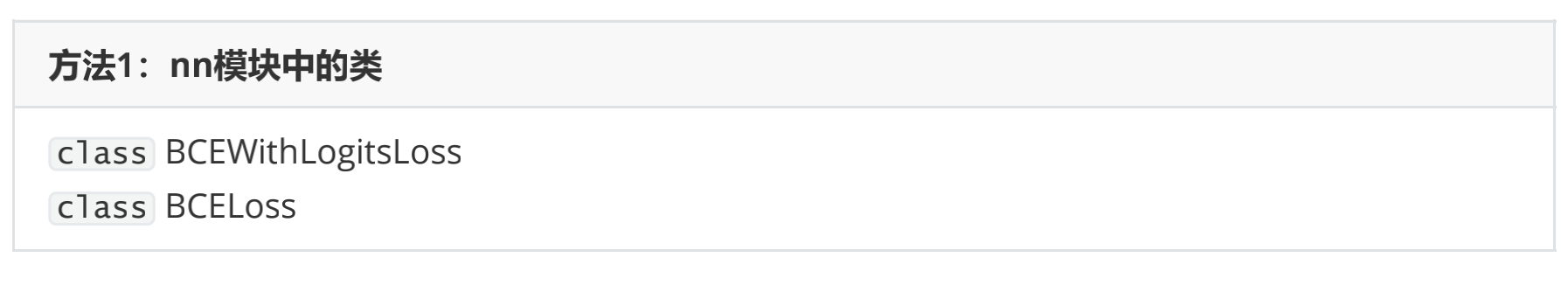对于二分类交叉熵损失，nn提供了两个类：BCEWithLogitsLoss以及BCELoss。虽然PyTorch官方没有直接明确，但实际上两个函数所需要输入的参数不同。

BCEWithLogitsLoss内置了sigmoid函数与交叉熵函数，它会自动计算输入值的sigmoid值，因此需要输入zhat与真实标签，且顺序不能变化，zhat必须在前。

相对的，BCELoss中只有交叉熵函数，没有sigmoid层，因此需要输入sigma与真实标签，且顺序不能变化。

同时，这两个函数都要求预测值与真实标签的数据类型以及结构（shape）必须相同，否则运行就会报错。

接下来，我们来看看这两个类是如何使用的：

import torch.nn as nn
#调用nn模块下的类
criterion = nn.BCELoss() #实例化
loss = criterion(sigma,y) #真实标签在后
loss

criterion2 = nn.BCEWithLogitsLoss() #实例化
loss = criterion2(zhat,y) #真实标签在后
loss


可以看出，两个类的结果是一致的。根据PyTorch官方的公告，他们更推荐使用BCEWithLogitsLoss这个内置了sigmoid函数的类。内置的sigmoid函数可以让精度问题被缩小（因为将指数运算包含在了内部），以维持算法运行时的稳定性，即是说当数据量变大、数据本身也变大时，BCELoss类产生的结果可能有精度问题。所以，当我们的输出层使用sigmoid函数时，我们就可以使用BCEWithLogitsLoss作为损失函数

与MSELoss相同，二分类交叉熵的类们也有参数reduction，默认是”mean“，表示求解所有样本平均的损失，也可换为”sum”，要求输出整体的损失。以及，还可以使用选项“none”，表示不对损失结果做任何聚合运算，直接输出每个样本对应的损失矩阵。

criterion2 = nn.BCEWithLogitsLoss(reduction = "mean")
loss = criterion2(zhat,y)
loss

criterion2 = nn.BCEWithLogitsLoss(reduction = "sum")
loss = criterion2(zhat,y)
loss

criterion2 = nn.BCEWithLogitsLoss(reduction = "none")
loss = criterion2(zhat,y)
loss
#tensor([[1.3102],
#        [0.3155],
#        [0.4247],
#        ...,
#        [0.1727],
#        [0.1716],


第二种方法很少用，我们了解一下即可：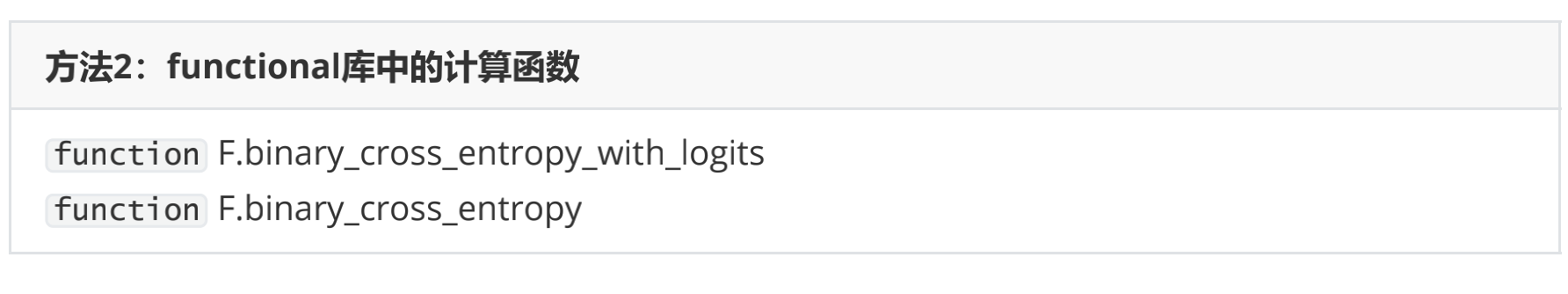和nn中的类们相似，名称中带有Logits的是内置了sigmoid功能的函数，没有带Logits的，是只包含交叉熵损失的函数。对于含有sigmoid功能的函数，我们需要的输入是zhat与标签，不含sigmoid的函数我们则需要输入sigma与标签。同样的，这两个函数对输入有严格的要求，输入的预测值必须与标签结构一致、数据类型一致。我们来看看他们的运行结果：

from torch.nn import functional as F #直接调用functional库中的计算函数
F.binary_cross_entropy_with_logits(zhat,y)

F.binary_cross_entropy(sigma,y)


在这里，两个函数的运行结果是一致的。同样的，PyTorch官方推荐的是内置sigmoid功能的函数binary_cross_entropy_with_logits。通常来说，我们都使用类，不使用函数。虽然代码会因此变得稍稍有点复杂，但为了代码的稳定性与日后维护，使用类是更好的选择。当然，在进行代码演示和快速测算的时候，使用函数或者类都没有问题。

## 四、多分类交叉熵损失函数

### 1 由二分类推广到多分类

二分类交叉熵损失可以被推广到多分类上，但在实际处理时，二分类与多分类却有一些关键的区别。依然使用极大似然估计的推导流程，首先我们来确定单一样本概率最大化后的似然函数。

对于多分类的状况而言，标签不再服从伯努利分布（0-1分布），因此我们可以定义，样本i在由特征向量 x i x_{i} 和权重向量 w w 组成的预测函数中，样本标签被预测为类别k的概率为：
P k = P ( y ^ i = k ∣ x i , w ) = σ P_{k}=P\left(\hat{y}_{i}=k \mid \boldsymbol{x}_{i}, \boldsymbol{w}\right)=\sigma
对于多分类算法而言， 就是softmax函数返回的对应类别的值。

假设一种最简单的情况：我们现在有三分类[1, 2, 3]，则样本i被预测为三个类别的概率分别为：假设样本的真实标签为1，我们就希望 P 1 P_{1} 最大，同理，如果样本的真实标签为其他值，我们就希望其他值所对应的概率最大。在二分类中，我们将y和1-y作为概率 P P 的指数，以此来融合真实标签为0和为1的两种状况。但在多分类中，我们的真实标签可能是任意整数，无法使用y和1-y这样的结构来构建似然函数。所以我们认为，如果多分类的标签也可以使用0和1来表示就好了，这样我们就可以继续使用真实标签作为指数的方式。

因此，我们对多分类的标签做出了如下变化：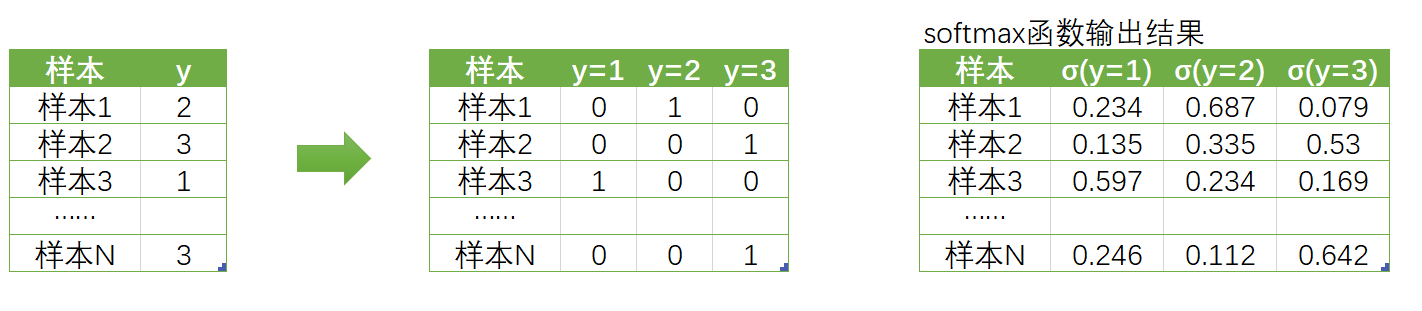原本的真实标签y是含有[1, 2, 3]三个分类的列向量，现在我们把它变成了标签矩阵，每个样本对应一个向量。（如果你熟悉机器学习或统计学，你能够一眼看出这其实就是独热编码one-hot）。在矩阵中，每一行依旧对应样本，但却由三分类衍生出了三个新的列，分别代表：真实标签是否等于1、等于2以及等于3。在矩阵中，我们使用“1”标注出样本的真实标签的位置，使用0表示样本的真实标签不是这个标签。不难注意到，这个标签矩阵的结构其实是和softmax函数输出的概率矩阵的结构一致，并且一一对应的。

回顾下二分类的似然函数：
P ( y ^ i ∣ x i , w ) = P 1 y i ∗ P 0 1 − y i P\left(\hat{y}_{i} \mid \boldsymbol{x}_{i}, \boldsymbol{w}\right)=P_{1}^{y_{i}} * P_{0}^{1-y_{i}}
当我们把标签整合为标签矩阵后，我们就可以将单个样本在总共K个分类情况整合为以下的似然函数：
P ( y ^ i ∣ x i , w ) = P 1 y i ( k = 1 ) ∗ P 2 y i ( k = 2 ) ∗ P 3 y i ( k = 3 ) ∗ … ∗ P K y i ( k = K ) P\left(\hat{y}_{i} \mid \boldsymbol{x}_{i}, \boldsymbol{w}\right)=P_{1}^{y_{i(k=1)}} * P_{2}^{y_{i(k=2)}} * P_{3}^{y_{i(k=3)}} * \ldots * P_{K}^{y_{i(k=K)}}
其中P就是样本标签被预测为某个具体值的概率，而右上角的指数就是标签矩阵中对应的值，即这个样本的真实标签是否为当前标签的判断（是就是1，否就是0）。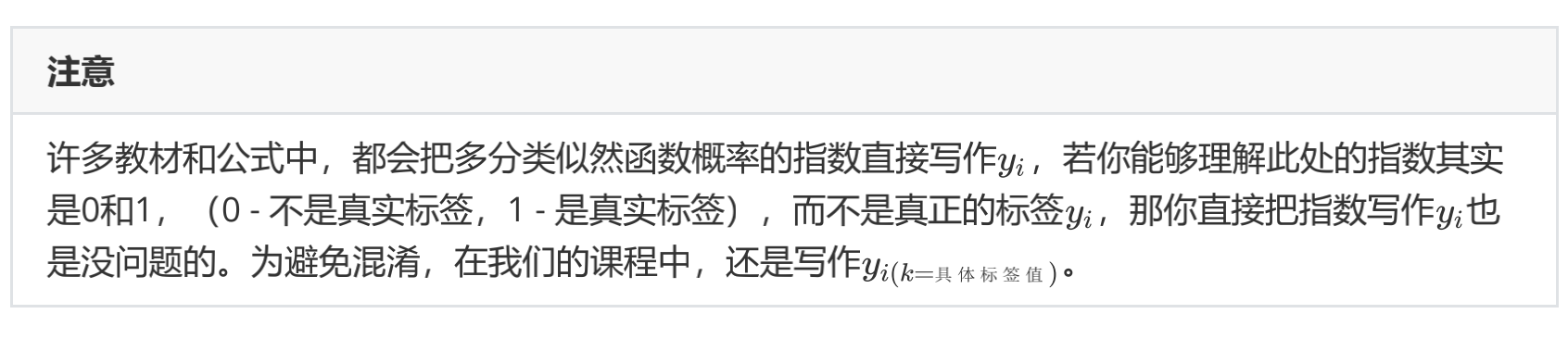更具体的，小k代表y的真实取值，K代表总共有K个分类（此处不是非常严谨，按道理说若K代表总共有K个类别，则不应该再使用K代表某个具体类别，但在这里，由于我们使用的类别编号与类别本身相同，所以为了公式的简化，使用了这样不严谨的表示方式）。虽然是连乘，但对于一个样本，除了自己所在的真实类别指数会是1之外，其他类别的指数都为0，所以被分类为其他类别的概率在这个式子里就都为0。所以我们可以将式子简写为：
P ( y ^ i ∣ x i , w ) = P j y i ( k = j ) , j  为样本  i  所对应的真实标签的编号  P\left(\hat{y}_{i} \mid \boldsymbol{x}_{i}, \boldsymbol{w}\right)=P_{j}^{y_{i(k=j)}}, j \text { 为样本 } i \text { 所对应的真实标签的编号 }
对一个训练集的m个样本来说，我们可以定义如下等式来表达所有样本在特征张量 X X 和权重向量 w w 组成的预测函数中，预测出所有可能的 y ^ \hat{y} 的概率P为：
P = ∏ i = 1 m P ( y ^ i ∣ x i , w ) = ∏ i = 1 m P j y i ( k = j ) = ∏ i = 1 m σ j y i ( k = j ) \begin{aligned} \boldsymbol{P} &=\prod_{i=1}^{m} P\left(\hat{y}_{i} \mid x_{i}, w\right) \\ &=\prod_{i=1}^{m} P_{j}^{y_{i(k=j)}} \\ &=\prod_{i=1}^{m} \sigma_{j}^{y_{i(k=j)}} \end{aligned}
这就是多分类状况下的似然函数。与二分类一致，似然函数解出来后，我们需要对似然函数求对数：
ln ⁡ P = ln ⁡ ∏ i = 1 m σ j y i ( k − j ) = ∑ i = 1 m ln ⁡ ( σ j y i ( k = j ) ) = ∑ i = 1 m y i ( k = j ) ln ⁡ σ i \begin{aligned} \ln \boldsymbol{P} &=\ln \prod_{i=1}^{m} \sigma_{j}^{y_{i(k-j)}} \\ &=\sum_{i=1}^{m} \ln \left(\sigma_{j}^{y_{i(k=j)}}\right) \\ &=\sum_{i=1}^{m} y_{i(k=j)} \ln \sigma_{i} \end{aligned}
这个函数就是我们之前提到过的交叉熵函数。不难看出，二分类的交叉熵函数其实是多分类的一种特殊情况。交叉熵函数十分特殊，虽然我们求解过程中，取对数的操作是在确定了似然函数后才进行的，但从计算结果来看，对数操作其实只对softmax函数的结果 σ \sigma 起效。因此在实际操作中，我们把 ln ⁡ ( softmax ⁡ ( z ) ) \ln (\operatorname{softmax}(z)) 这样的函数单独定义了一个功能做logsoftmax，PyTorch中可以直接通过nn.logsoftmax类调用这个功能。同时，我们把对数之外的，乘以标签、加和、取负等等过程打包起来，称之为负对数似然函数（Negative Log Likelihood function），在PyTorch中可以使用nn.NLLLoss来进行调用。也就是说，在计算损失函数时，我们不再需要使用单独的softmax函数了。

### 2 用PyTorch实现多分类交叉熵损失

在PyTorch中实现交叉熵函数的时候，有两种办法：

• 调用logsoftmax和NLLLoss实现
import torch
import torch.nn as nn
N = 3*pow(10,2)
torch.random.manual_seed(420)
X = torch.rand((N,4),dtype=torch.float32)
#定义y时应该怎么做？应该设置为矩阵吗？
y = torch.randint(low=0,high=3,size=(N,),dtype=torch.float32)
zhat = torch.mm(X,w) #从这里开始调用softmax和NLLLoss

logsm = nn.LogSoftmax(dim=1) #实例化
logsigma = logsm(zhat)

criterion = nn.NLLLoss() #实例化
#由于交叉熵损失需要将标签转化为独热形式，因此不接受浮点数作为标签的输入
#对NLLLoss而言，需要输入logsigma
criterion(logsigma,y.long()) #y一维、整型


更加简便的方法是：

• 直接调用CrossEntropyLoss
criterion = nn.CrossEntropyLoss()
criterion(zhat,y.long()) #一维、整型


可以发现，两种输出方法得到的损失函数结果是一致的。与其他损失函数一致，CrossEntropyLoss也有参数reduction，可以设置为mean、sum以及None，大家可以自行尝试其代码并查看返回结果。

无论时二分类还是多分类，PyTorch都提供了包含输出层激活函数和不包含输出层激活函数的类两种选择。在实际神经网络建模中，类可以被放入定义好的Model类中去构建神经网络的结构，因此是否包含激活函数，就需要由用户来自行选择。

• 重视展示网络结构和灵活性，应该使用不包含输出层激活函数的类

通常在Model类中，__init__中层的数量与forward函数中对应的激活函数的数量是一致的，如果我们使用内置sigmoid/logsoftmax功能的类来计算损失函数，forward函数在定义时就会少一层（输出层），网络结构展示就不够简单明了，对于结构复杂的网络而言，结构清晰就更为重要。同时，如果激活函数是单独写的，要修改激活函数就变得很容易，如果混在损失函数中，要修改激活函数时就得改掉整个损失函数的代码，不利于维护。

• 重视稳定性和运算精度，使用包含输出层激活函数的类

如果在一个Model中，很长时间我们都不会修改输出层的激活函数，并且模型的稳定运行更为要紧，我们就使用内置了激活函数的类来计算损失函数。同时，就像之前提到的，内置激活函数可以帮助我们推升运算的精度。

因此，选择哪种损失函数的实现方式，最终还要看我们的需求。

有了损失函数，我们终于要开始进行求解了。下一部分我们来讲解神经网络的入门级优化算法：小批量随机梯度下降。

展开全文分类 神经网络 机器学习
• 二分类问题的交叉熵损失函数;在二分类问题中，损失函数为交叉熵损失函数。对于样本(x,y)来讲，x为样本 y为对应的标签。在二分类问题中，其取值的集合可能为{0，1}，我们假设某个样本的真实标签为yt，该样本的yt=1的...
• 交叉熵：物理意义： 用模拟分布Q去编码真实分布P所需要的平均编码长度(比特个数底数为2时交叉熵损失函数:(这里的连加sigma 是把一个样本分布的所有概率下的信息量进行的连加，例如我们就求一句话分类的损失， 假设...
• 本代码基于pytorch实现，复现ICCV 2019论文 Robust Loss Functions under Label Noise for Deep Neural Networks，论文地址https://arxiv.org/abs/1712.09482v1
• 本文参照torch.nn.CrossEntropyLoss()说明文档1，从原理和实现细节上对交叉熵损失进行深入理解。 一、交叉熵 1.1 交叉熵的定义 假设X是离散型随机变量，p(x)、q(x)p(x)、q(x)p(x)、q(x)为X的两个概率分布，交叉熵的...机器学习 算法
• 在深度学习领域出现交叉熵（cross entropy）的地方就是交叉熵损失函数了。通过交叉熵来衡量目标与预测值之间的差距。了解交叉熵还需要从信息论中的几个概念说起。 信息量 如何衡量一条信息包含的信息量？加入我们有...自然语言处理 人工智能 nlp
• ## 史上最全交叉熵损失函数详解

千次阅读 多人点赞 2022-01-02 09:57:10
在我们自学神经网络神经网络的损失函数的时候会发现有一个思路就是交叉熵损失函数，交叉熵的概念源于信息论，一般用来求目标与预测值之间的差距。比如说我们在人脑中有一个模型，在神经网络中还有一个模型，我们需要...python 开发语言 后端
• 1、提到二分类问题容易想到逻辑回归算法，而逻辑回归算法最关键的步骤就是将线性模型输出的实数域映射到[0, 1]表示概率分布的有效实数空间，其中Sigmoid函数和softmax函数刚好具有这样的功能。 2、Sigmoid函数 当...python
• 交叉熵函数直观理解三.交叉熵函数求导3.1Sigmoid + Cross-entropy3.2SoftMax + Cross-entropy四.交叉熵与SoftMax函数 一.交叉熵函数的由来(推导) 我们一共有m组已知样本, (x(i),y(i))(x^{(i)},y^{(i)})(x(i),y(i))...机器学习
• 进行二分类或多分类问题时，在众多损失函数中交叉熵损失函数较为常用。 下面的内容将以这三个问题来展开 写目录标题什么是交叉熵损失以图片分类问题为例，理解交叉熵损失函数从0开始实现交叉熵损失函数 什么是交叉熵...机器学习
• 六、损失函数交叉熵 机器学习中，实际分布为p，预测分布为q。训练的过程就是调整参数使q不断逼近p的过程。调整的依据就是q与p的差。 如何衡量这个差呢？ 就可以用相对熵： 但由于实际分布的熵： 固定不变。 所以...
• 说起交叉熵损失函数「Cross Entropy Loss」，相信大家都非常熟悉，但是要深入理解交叉熵损失函数的原理和作用，还得溯本追源才能对其有一个真实的理解与认知。 交叉熵 交叉熵是用来度量两个概率分布的差异性的，...信息熵 机器学习ML...